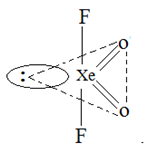Courses

# Notes | EduRev

## JEE : Notes | EduRev

The document Notes | EduRev is a part of the JEE Course JEE Revision Notes.
All you need of JEE at this link: JEE

Question 1: Stability of the species Li2, Li2- , Li2+ increases in the order of:    (IIT JEE 2013)
(a) Li2- < Li2+ < Li2
(b) Li< Li2- < Li2+
(c) Li2- < Li< Li2+
(d) Li< Li2+ < Li2-
Solution:
Stability of species depends on its bond order. Greater the bond order, more stable would be the species.
Electronic configuration of Li2 = σ1s2, σ*1s2, σ2s2
Bond Order = (No. of electrons in bonding orbitals –No. of electrons in antibonding orbitals)/2
= ½ (4-2) =1
Electronic configuration of Li2+ = σ1s2, σ*1s2, σ2s1
Bond Order = ½(3-2) = 0.5
Electronic configuration of Li2- = σ1s2, σ*1s2, σ2s2, σ*2s1
Bond Order = ½(4-3) = 0.5
Bond order of both Li2+ and Li2- is same but  Li2+ will be more stable as it has lesser number of antibonding electrons.
So, the correct order is Li2< Li2+ < Li2
And hence, the correct order is a.

Question 2: Which one of the following molecules is expected to exhibit diamagnetic behaviour?    (IIT JEE 2013)
(a) N2
(b) O2
(c) S2
(d) C2

N2(14) = σ1s2, σ*1s2, σ2s2, σ*2s2, σ2p6
There is no unpaired electron so it is diamagnetic.
O2(16) = s1s2, s* 1s2, s2s2 , s*2s2, s2p2x , p2py2  , p2pz2, p*2py1  , p2pz1
There are two unpaired electrons in antibonding 2p orbitals, hence it is paramagnetic.
C2(12) = σ1s2, σ*1s2, σ2s2, σ*2s2, p2py2 , p2pz2
There are no unpaired electrons, hence it is diamagnetic.
S2(32) = s1s2, s* 1s2, s2s2 , s*2s2, s2p2, p2py2  , p2pz2, p*2py2  , p2pz2 , s*2p2x  s3s2 , s*3s2, s3p2x , p2py2  , p2pz2, p*2py1  , p2pz1
There are two unpaired electrons in antibonding 3p orbitals, hence it is paramagnetic.
Hence, b and c are the correct options.

Question 3: The shape of XeO2Fmolecule is (IIT JEE -2012)
(a) trigonal bipyramidal
(b) square planar
(c) tetrahedral
(d) see-saw
Solution:
XeO2Fmolecule has see-saw geometry with two F atoms on axial positions while two O atoms and a lone pair of electrons on equatorial positions.Hence, the correct option is d.

Offer running on EduRev: Apply code STAYHOME200 to get INR 200 off on our premium plan EduRev Infinity!

233 docs

,

,

,

,

,

,

,

,

,

,

,

,

,

,

,

,

,

,

,

,

,

;